## Worksheet 03.2: Descriptive Measures

The task here is to come up with descriptive measures for the data in Table 1.

 Table 1 Item number 1 2 3 4 5 6 6 8 9 10 Data value 34 37 38 41 42 43 46 47 51 53 Frequency of data 8 11 2 7 13 17 5 11 15 8

This page assumes that you have read through earlier pages and that you have mastered the steps that we use to set up our work. To that end we will assume that we have
1. inserted our USB drive,
2. created a directory called `worksheet032` on that drive
3. have copied `model.R` from our root folder into our new folder,
4. have renamed that new copy of the file to the name `ws32.R`, and
5. have double clicked on that file to open RStudio.
The result should be a window pretty much identical to the one shown in Figure 1. {Recall that the images shown here may have been reduced to make a printed version of this page a bit shorter than it woud otherwise appear. In most cases your browser should allow you to right click on an image and then select the option to View Image in order to see the image in its original form.}

Figure 1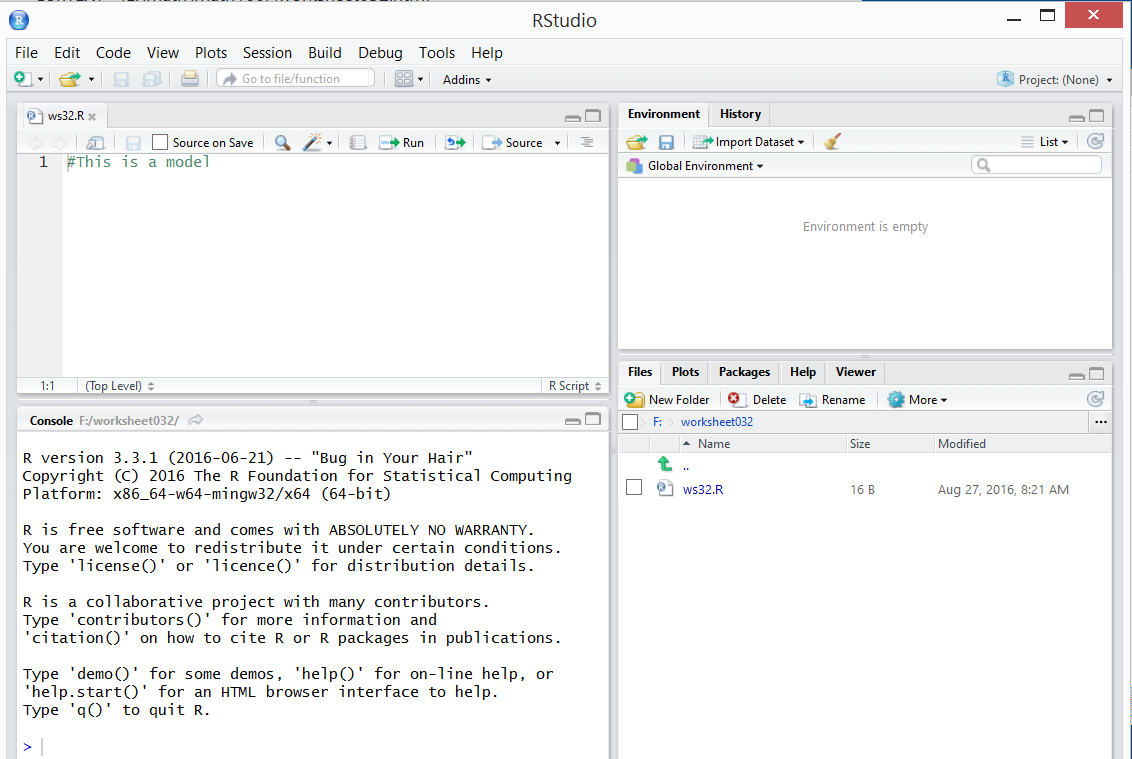The data that we have been given in Table 1 shows us the frequency of each of the different data values. Rather than try to work with that consolidated set of values we would prefer to work with the "raw" data. That is, we would like the data to hold 8 of the 34's, 11 of the 37's, 2 of the 38's, and so on. To do this we start by getting the different values and the different frequencies into R. The commands
```data_val <- c(34, 37, 38, 41, 42, 43, 46, 47, 51, 53)
data_freq <- c(8, 11,  2,  7, 13, 17,  5, 11, 15,  8)
```
will do this.

Figure 2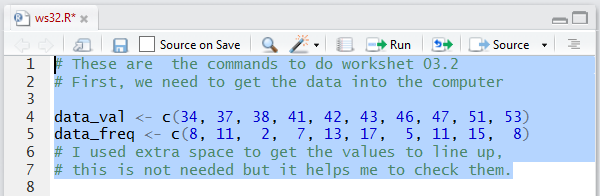When we run those commands the Console pane merely shows the the commands (and the comments).

Figure 3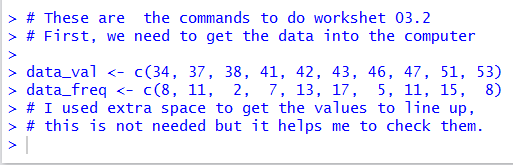However, if we look in the Environment pane we can see that our two new variables have been created and we can see that they hold the correct values (well, actually all we see is that `data_freq` holds the correct 10 values, but the pane shown in Figure 4 is only wide enough to show the first 8 values in `data_val`.

Figure 4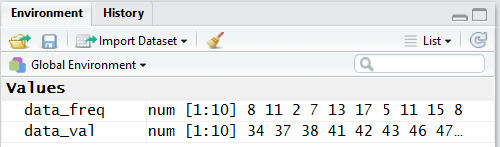Then, we use those two variables in the `rep` function to produce all of the desired values and store them in the variable `data_raw`.

Figure 5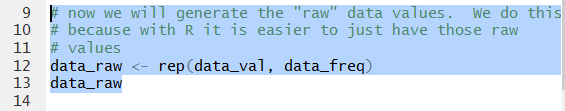The Console display shown in Figure 6 shows our 97 values comprised of eight 34's, eleven 37's, two 38's, and so on.

Figure 6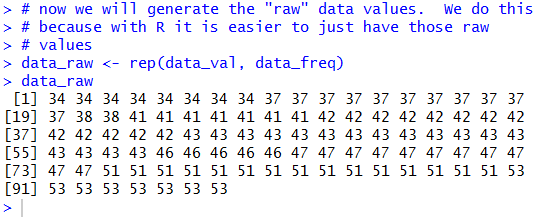Figure 7 shows the new variable in the Environment pane.

Figure 7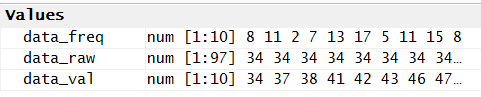Of course, now that we have the 97 raw values we can just process them sas we usually do. Figure 8 holds the commands to get a `summary` of the values, then to compute and display the `mean` separately, then to find the standard deviation assuming Table 1 represents a sample, then loading the `pop_sd` function into our environment, and finally, using the `pop_sd` function to find the standard deviation assuming the data in Table 1 represents a population.

Figure 8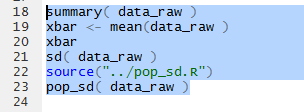Figure 9 shows the Console output from those commands. Again, we notice the brevity of the display of the value of the mean by the `summary` function and the increase in the number of digits displayed by computing the mean separately and displaying it.

Figure 9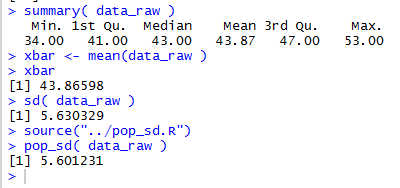Examining the Environment pane we see the display of the value there has even more significant digits.

Figure 10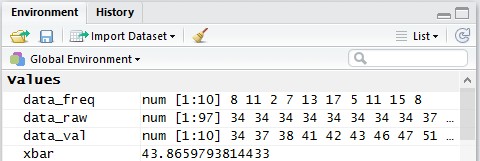The appropriate graph for this kind of data is a bar chart. Figure 11 shows the command that we would use to get the default graphic.

Figure 11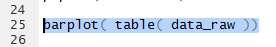Running that command will produce the graph shown in Figure 12.

Figure 12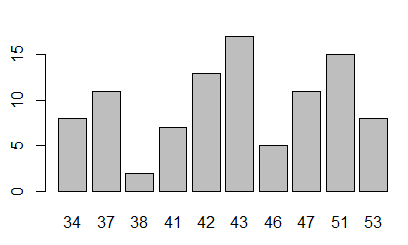There is nothing wrong about the graph shown in Figure 12, but we have seen, on other pages, some of the commands that we can use to improve the appearance of that chart. Figure 13 shows the more elaborate commands.

Figure 13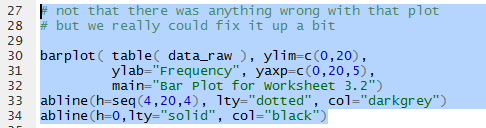Running the commands of Figure 13 gives the graph shown in Figure 14. This is more informative and it is easier to read.

Figure 14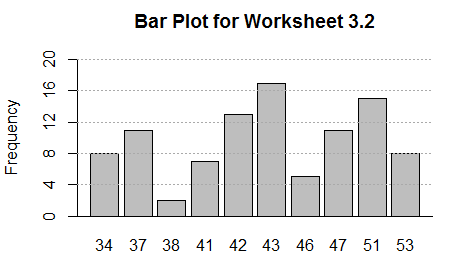We could also produce a frequency table for the data. It is interesting to note that Table 1 really stated the first part of such a frequency table. However, because we have created the raw data and because we have the function `make_freq_table` (which we have on the USB drive but which we need to load into our environment, the command that we use
```make_freq_table( data_raw )
```
looks at `data_raw` and processes the values in it to get back to Table 1 and then to expand it with values for the relative frequency, the cumulative frequency, the relative cumulative frequency, and the number of degrees required for ach value in a pie chart.

Figure 15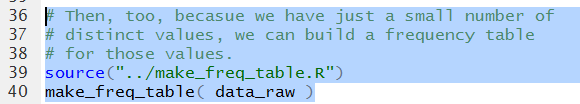Figure 16 shows the Console display of that frequency table.

Figure 16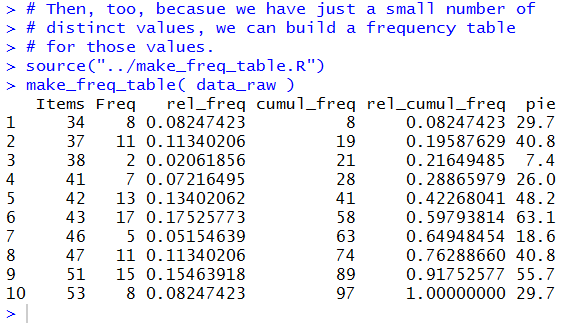The Console display version is OK, but we recall that we could get a much prettier display. To do that we will have R compute the table again but this time we will store the result, the frequency table, in a variable, in this case `data_ft`, and then we will `View` that variable. Note the capital V in the command `View(data_ft)`.

Figure 17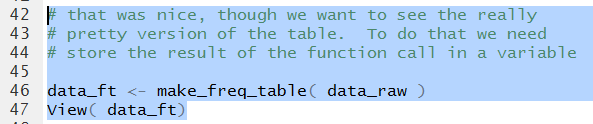Of course, running the new commands does little in the Console pane, shown in Figure 18.

Figure 18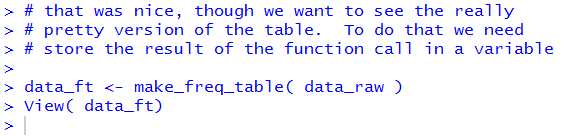However, it does open a new tab in the Editor pane shown in Figure 19.

Figure 19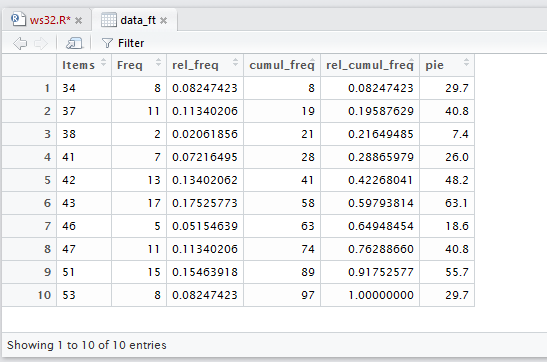Finally, before we leave all of this work, we note that our file, `ws32.R` has had its contents changed causing its name to appear in red in the tab.

Figure 20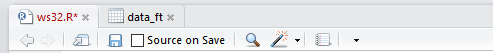We will click on the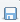to save that file. This will change the name to black letters.

Figure 21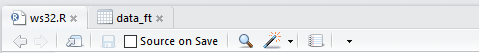Once that is done we move to the Console pane and directly enter the command `q()` k followed by the Enter key. Then we respond to the question with `y` as in Figure 22.

Figure 22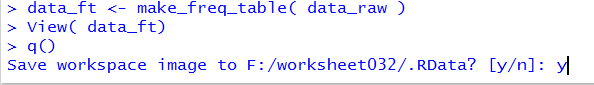Once we press Enter at that point RStudio will save our hidden files and terminate.
Here is a listing of the complete contents of the `ws32.R` file:
```# These are  the commands to do workshet 03.2
# First, we need to get the data into the computer

data_val <- c(34, 37, 38, 41, 42, 43, 46, 47, 51, 53)
data_freq <- c(8, 11,  2,  7, 13, 17,  5, 11, 15,  8)
# I used extra space to get the values to line up,
# this is not needed but it helps me to check them.

# now we will generate the "raw" data values.  We do this
# because with R it is easier to just have those raw
# values
data_raw <- rep(data_val, data_freq)
data_raw

# now that we have the "raw" data we can go ahead and
# get our usual measures

summary( data_raw )
xbar <- mean(data_raw )
xbar
sd( data_raw )
source("../pop_sd.R")
pop_sd( data_raw )

barplot( table( data_raw ))

# not that there was anything wrong with that plot
# but we really could fix it up a bit

barplot( table( data_raw ), ylim=c(0,20),
ylab="Frequency", yaxp=c(0,20,5),
main="Bar Plot for Worksheet 3.2")
abline(h=seq(4,20,4), lty="dotted", col="darkgrey")
abline(h=0,lty="solid", col="black")

# Then, too, becasue we have just a small number of
# distinct values, we can build a frequency table
# for those values.
source("../make_freq_table.R")
make_freq_table( data_raw )

# that was nice, though we want to see the really
# pretty version of the table.  To do that we need
# store the result of the function call in a variable

data_ft <- make_freq_table( data_raw )
View( data_ft)
```